Courses

# ICAI Notes 2.3 - Law of Supply CA Foundation Notes | EduRev

## CA Foundation : ICAI Notes 2.3 - Law of Supply CA Foundation Notes | EduRev

The document ICAI Notes 2.3 - Law of Supply CA Foundation Notes | EduRev is a part of the CA Foundation Course Economics for CA CPT.
All you need of CA Foundation at this link: CA Foundation

Learning Objectives

understand the meaning of supply.
understand what determines supply.
get an insight into the law of supply.
know the difference between movements on the supply curve and shift of the supply curve.
understand the concept of elasticity of supply.

3.0 INTRODUCTION

As the term ‘demand’ refers to the quantity of a good or service that the consumers are willing and able to purchase at various prices during a period of time, the term ‘supply’ refers the amount of a good or service that the producers are willing and able to offer to the market at various prices during a period of time. Two important points apply to supply :

(i) The supply refers to what firms offer for sale, not necessarily to what they succeed in selling.

(ii) Supply is a flow. The quantity supplied is so much per unit of time, per day, per week, or per year.

Supply is defined as “how much of good will be offered for sale at a given time”. Prof. McConnell defines supply in the following term: “Supply may be defined as a schedule which shows the various amounts of a product which a producer is willing to and able to produce and make available for sale in the market at each specific price in a set of possible prices during some given period”.

3.1 DETERMINANTS OF SUPPLY

Although price is an important consideration in determining the willingness and desire to part with the commodities, they are many other factors which determine the supply of a product or a service. These are discussed below :

(i) Price of the good : Other things being equal, the higher the relative price of a good the greater the quantity of it that will be supplied. This is because goods and services are produced by the firm in order to earn profits and, ceteris paribus, profits rise if the price of its product rises.

(ii) Price of the related goods : If the prices of other goods rise, they become relatively more profitable to the firm to produce and sell than the good in question. It implies, that if the price of Y rises, the quantity supplied of X will fall. For example, if price of wheat rises, the farmers may shift lands to wheat production and away from corn and soyabeans.

(iii) Price of the factors of production : A rise in the price of a particular factor of production will cause an increase in the cost of making those goods that use a great deal of that factor than in the costs of producing those that use relatively small amount of the factor. For example, a rise in the cost of land will have a large effect on the cost of producing wheat and a very small effect on the cost of producing automobiles. Thus a change in the price of one factor of production will cause changes in the relative profitability of different lines of production and will cause producers to shift from one line to another and thus supplies of different commodities will change.

(iv) State of technology : The supply of a particular product depends upon the state of technology also. Inventions and innovations tend to make it possible to produce more or better goods with the same resources, and thus they tend to increase the quantity supplied of some products and to reduce the quantity supplied of products that are displaced.

(v) Government Policy : The production of a good may be subject to the imposition of commodity taxes such as excise duty, sales tax and import duties. These raise the cost of production and so the quantity supplied of a good would increase only when its price in the market rises. Subsidies, on the other hand, reduce the cost of production and thus provide an incentive to the firm to increase supply. (vi) Other Factors : The quantity supplied of a good also depends upon government’s industrial and foreign policies, goals of the firm, infrastructual facilities, market structure, natural factors etc.

3.2 LAW OF SUPPLY

This refers to the relationship of quantity supplied of a good with one or more related variables which have an influence on the supply. Normally, the supply is related with price but it can be related with the type of technology used, scale of operations etc. The law of supply can be stated as : Other things remaining constant, the quantity of a good produced and offered for sale will increase as the price of the good rises and decrease as the price falls.

This law is based upon common sense, for the higher the price of the good, the greater the profits that can be earned and thus greater the incentives to produce the good and offer it for sale. The law is known to be correct in large number of cases. There is an exception however. If we take the supply of labour at very high wages, we may find that the supply of labour has decreased instead of increasing. Thus, the behaviour of supply depends upon the phenomenon considered and the degree of possible adjustment in supply.

The behaviour of supply curve is also affected by the time taken into consideration. In the short run, it may not be easy to increase supply but in the long run supply can be easily adjusted in response to changes in price. The law of supply can be explained through supply schedule and supply curve. Consider the following schedule.

Table 8 : Supply Schedule of Good ‘X’

 Price (Rs.) (per kg) Quantity supplied (kg) 1 5 2 35 3 45 4 55 5 65

The table shows the quantities of good X that would be produced and offered for sale at a number of alternative prices. At Re. 1, for example, 5 kilograms of good X are offered for sale and at Rs. 3 per kg. 45 kg. would be forthcoming.

We can now plot the data from Table 8 on a graph. In Figure 21, price is plotted on vertical axis and quantity on the horizontal axis, and various price-quantity combinations of the schedule 8 are plotted.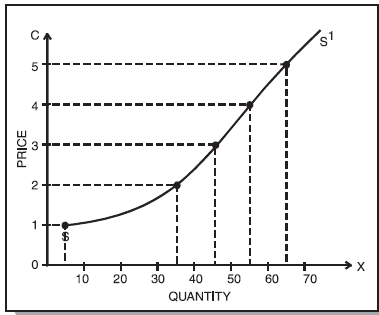Fig. 21 : Supply Curve

When we draw a smooth curve through the plotted points, what we get is the supply curve for good X. The curve shows the quantity of X that will be offered for sale at each price of X. It slopes upwards towards right showing that as price increases, the supply of X increases and vice-versa.

The market supply curve for ‘X’ can be obtained by adding horizontally the various firms’ supply curves.

3.3 SHIFTS IN THE SUPPLY CURVE – INCREASE OR DECREASE IN SUPPLY

When the supply curve bodily shifts towards right as a result of a change in one of the factors that influence the quantity supplied other than the commodity’s own price, we say there is an increase in supply. When these factors cause the supply curve to shift to left we call it decrease in supply [See Figures 22(i) and (ii)].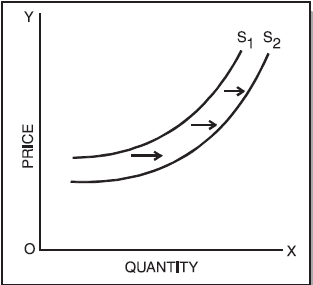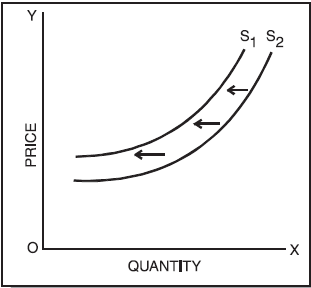Fig. 22 : Shifts in supply curves

3.4 MOVEMENTS ON THE SUPPLY CURVE – INCREASE OR DECREASE IN THE QUANTITY SUPPLIED

When the supply of a good increases as a result of an increase in its price we say that there is an increase in the quantity supplied and there is a upward movement on the supply curve. The reverse is the case when there is a fall in the price of the good. (See Figure 23).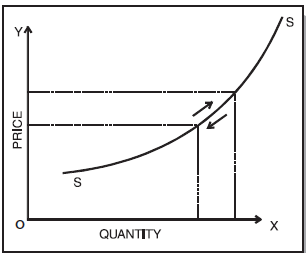Fig. 23 : Figure showing change in quantity supplied as a result of a price change

3.5 ELASTICITY OF SUPPLY

The elasticity of supply is defined as the responsiveness of the quantity supplied of a good to a change in its price. Elasticity of supply is measured by dividing the percentage change in quantity supplied of a good by the percentage change in its price i.e.,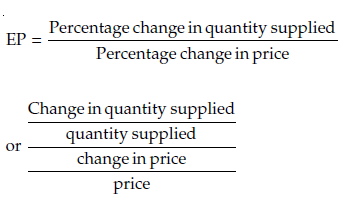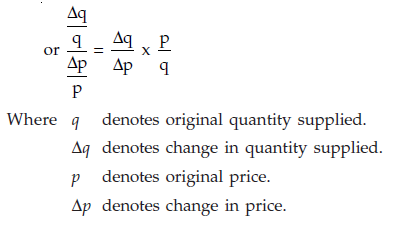Example:

a. Suppose the price of a commodity X increase from Rs. 2,000 per unit to Rs. 2,100 per unit and consequently the quantity supplied rises from 2,500 units to 3,000 units. Calculate the elasticity of supply.

Here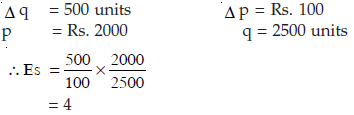Elasticity of Supply = 4.

3.5.0 Type of Supply Elasticity : The elasticity of supply can be classified as under :

(i) Perfectly Inelastic supply : If as a result of a change in price, the quantity supplied of a good remains unchanged, we say that the elasticity of supply is zero or the good has perfectly inelastic supply. The vertical supply curve in Figure 24 shows that irrespective of the price change, the quantity supplied remains unchanged.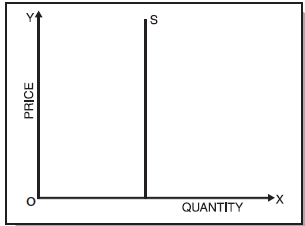Fig. 24 : Supply curves of zero elasticity

(ii) Relatively less-elastic supply : If as a result of a change in the price of a good its supply changes less than proportionately, we say that the good is relatively less elastic or elasticity of supply is less than one. Figure 25 shows that the relative change in the quantity supplied (Δq) is less than the relative change in the price (Δp).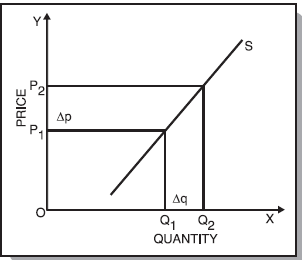Fig. 25 : Showing relatively less elastic supply

(iii) Relatively greater-elastic supply : If elasticity of supply is greater than one i.e., when the quantity supplied of a good changes substantially in response to a small change in the price of the good we say that supply in greatly elastic. Figure 26, shows that the relative change in the quantity supplied (Δq) is greater than the relative change in the price.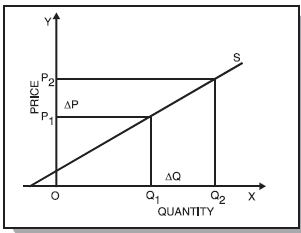Fig. 26 : Showing relatively greater elastic supply

(iv) Unit-elastic : If the relative change in the quantity supplied is exactly equal to the relative change in the price, the supply is said to be unitary elastic. Here coefficient of elasticity of supply is equal to one. In Figure 27, the relative change in the quantity supplied (Δq) is equal to the relative change in the price (Δp).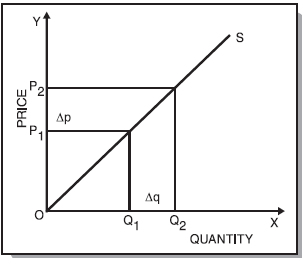Fig. 27 : Showing unitary elasticity

(v) Perfectly elastic supply : The supply elasticity is infinite when nothing is supplied at a lower price but a small increase in price causes supply to rise from zero to an indefinitely large amount indicating that producers will supply any quantity demanded at that price. Figure 28 shows infinitely elastic supply.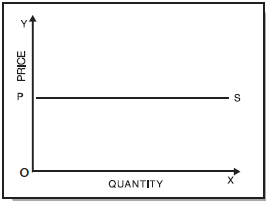Fig. 28 : Supply curve of infinite elasticity

3.5.1 Measurement of supply-elasticity : The elasticity of supply can be considered with reference to a given point on the supply curve or between two points on the supply curve.

Point-elasticity : Just as in demand, point-elasticity can be measured with the help of the following formula :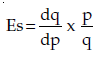(Ed) The Supply function is given as q = -100 + 10p. Find the elasticity of supply using point method, when price is Rs. 15.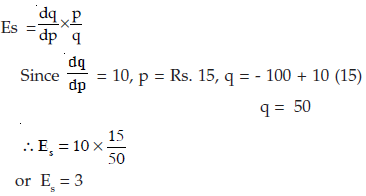Where dq/dp is differentiation of the supply function with respect to price and p and q refer to price and quantity respectively.

Arc-Elasticity : Arc-elasticity i.e. elasticity of supply between two prices can be found out with the help of the following formula :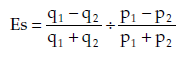Or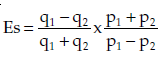Where p1 q1 are original price and quantity and p2 q2 are new price and quantity supplied. Thus, if we have to find elasticity of supply when p1 = Rs. 12, p2 = Rs. 15, q1 = 20 units and q2 = 50 units. Then using the above formula, we will get supply elasticity as :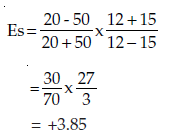SUMMARY

The term ‘Supply’ refers to a schedule of the quantities of a good that will be offered for sale at different prices. The supply curve is a graphic presentation of the supply schedule. The laws of supply explain the relation between the quantity supplied of a good or service and the various factors on which the supply depends like price of the product, technology used, scale of operations etc. Most important is the relation of the quantity supplied of a good with its price. It has been observed that quantity supplied of a good increases with a rise in its price and falls with a fall in its price.

Elasticity of supply is the responsiveness of quantity supplied of a good as a result of a change in any of the factors on which supply depends. Most important is the responsiveness of the quantity supplied to a change in the price of the good. Elasticity of supply can be considered with reference to a given point on the supply curve (point elasticity) or between two points (arc elasticity).

,

,

,

,

,

,

,

,

,

,

,

,

,

,

,

,

,

,

,

,

,

;